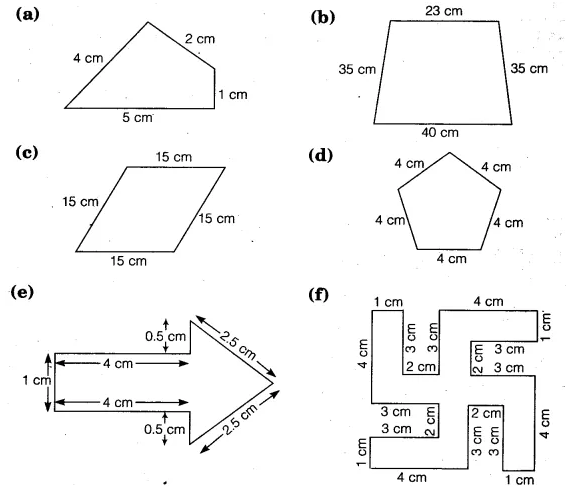# Find the perimeter of each of the following figures:

Find the perimeter of each of the following figures: